# 文章详细页

## [梅花藻]不把开花信息&折成的旅途的实施！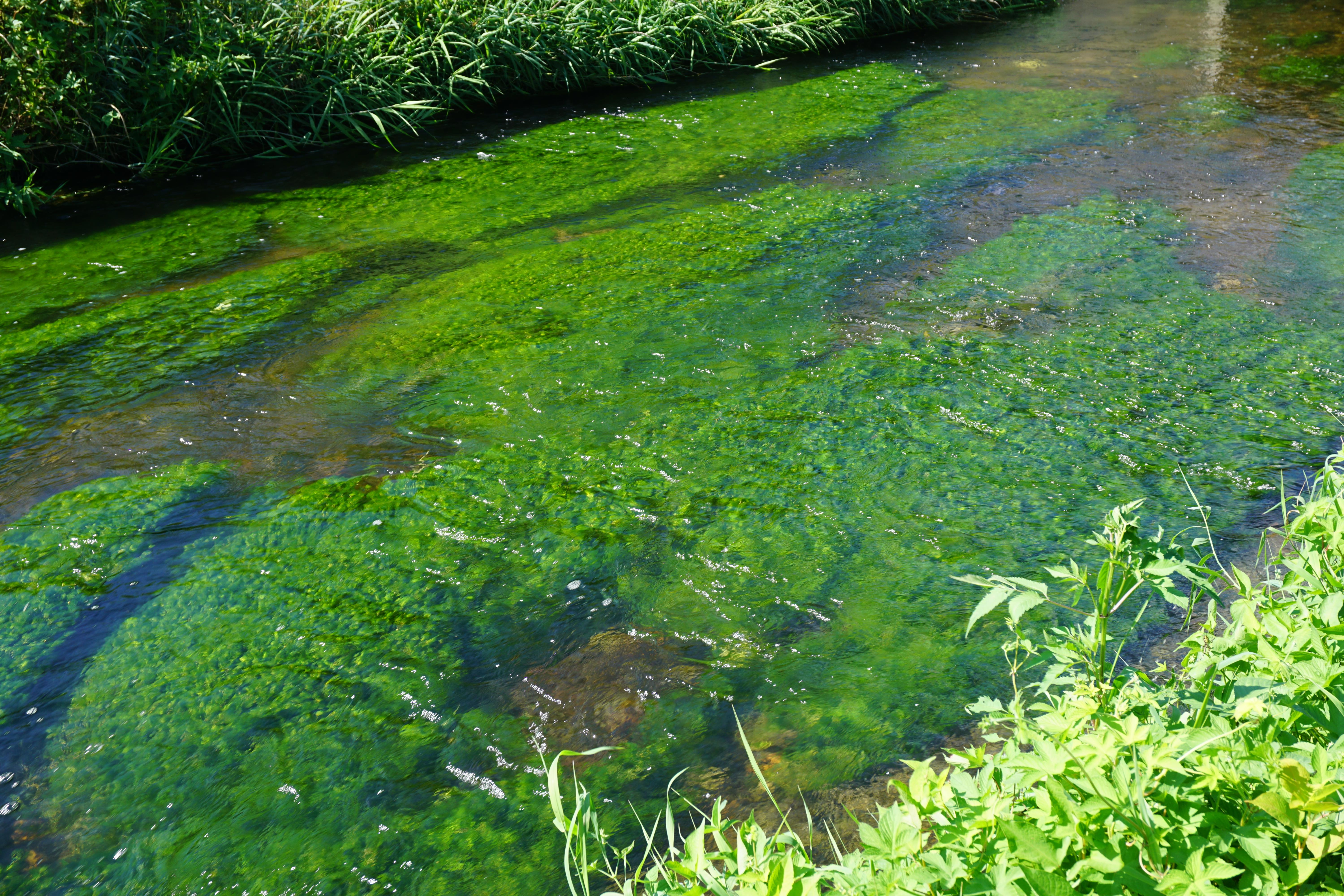(神社船港口附近)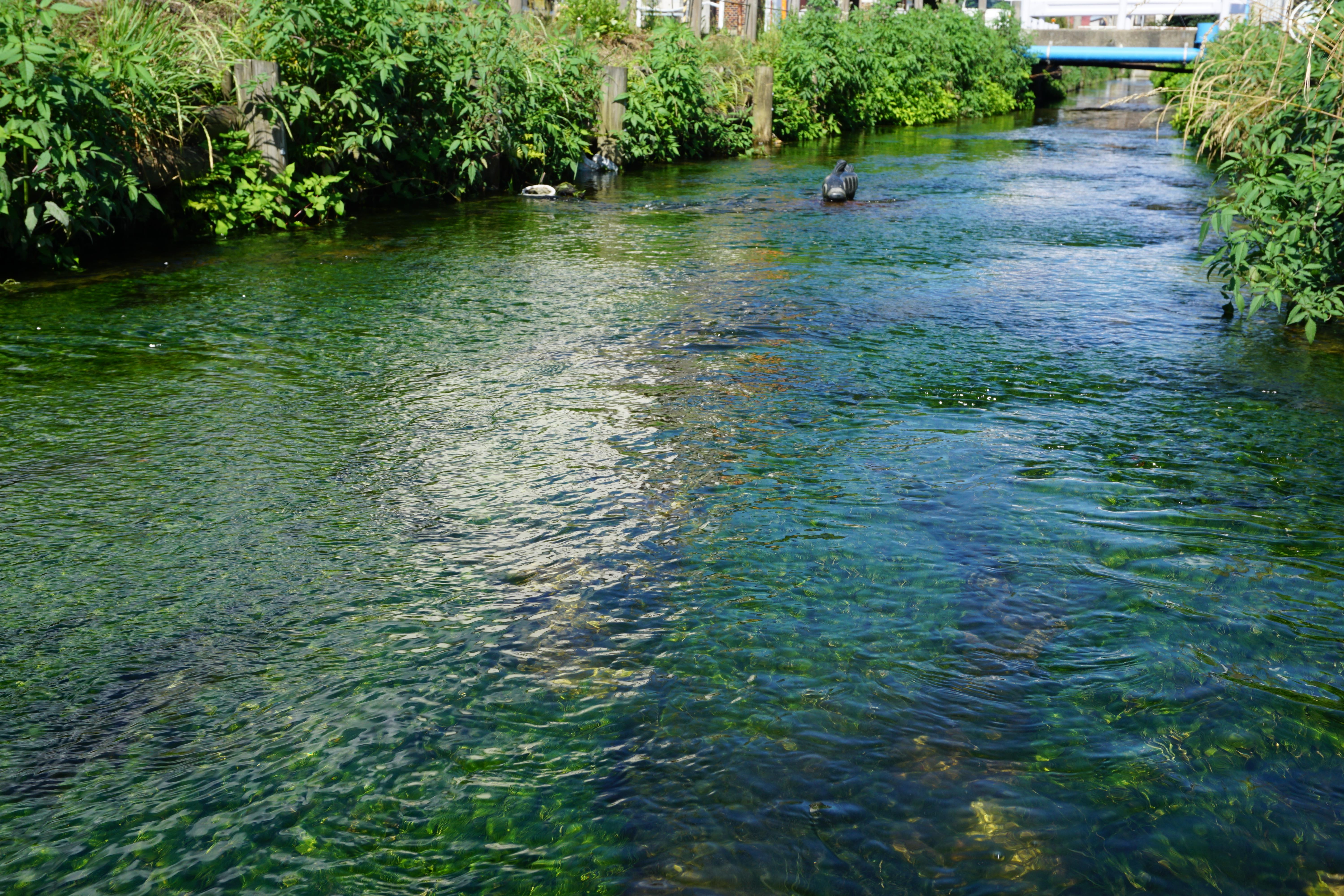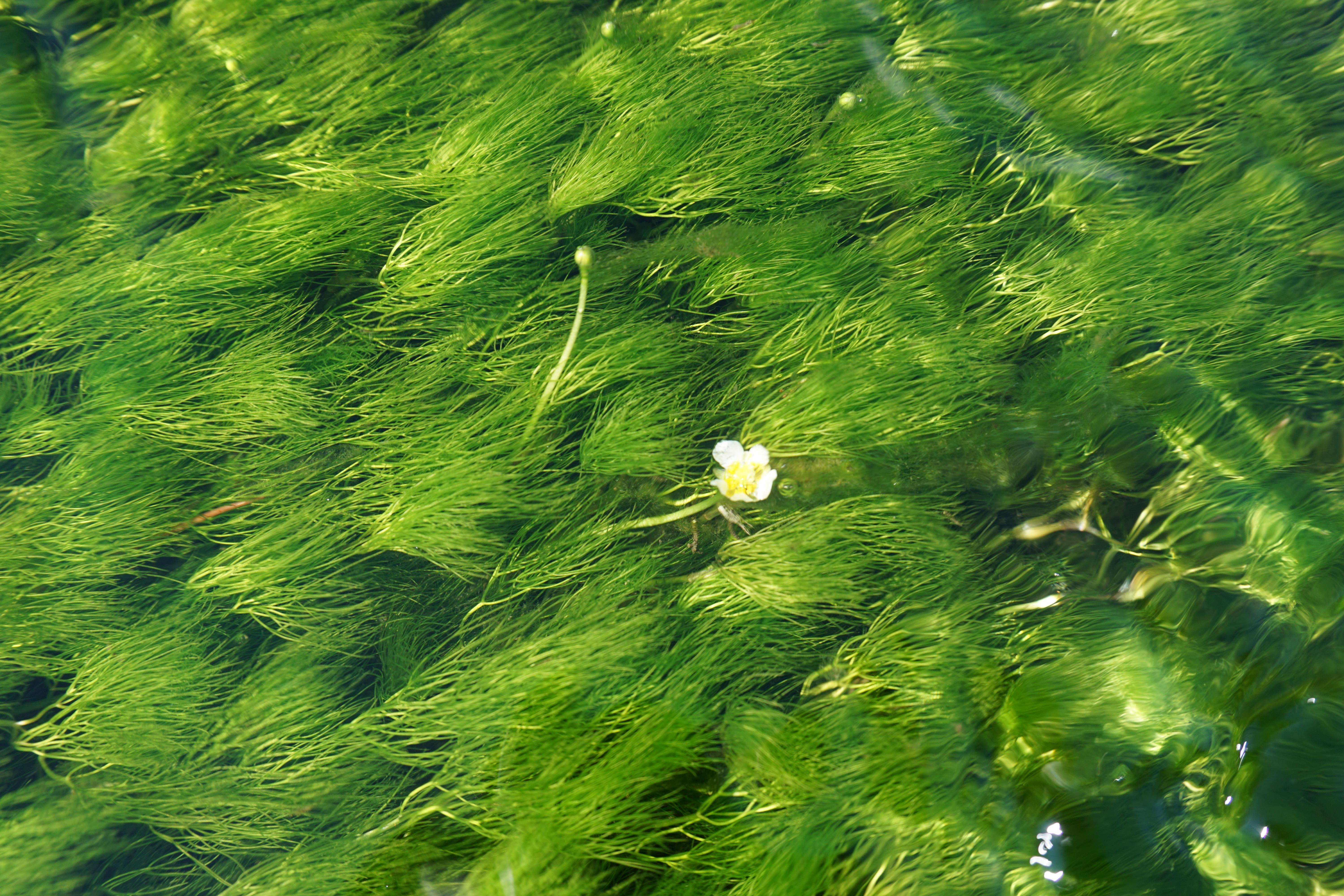(撞木川小溪水路)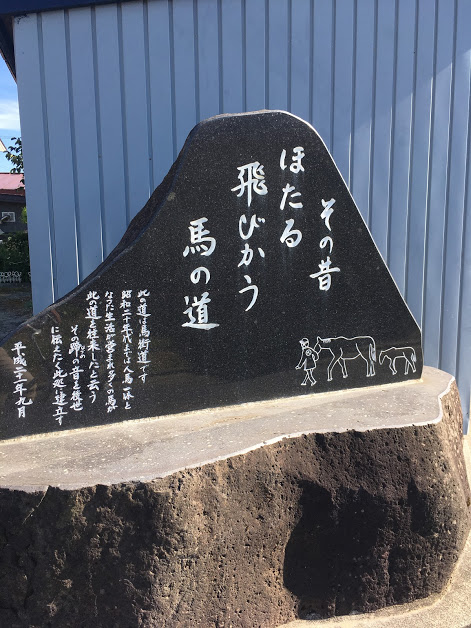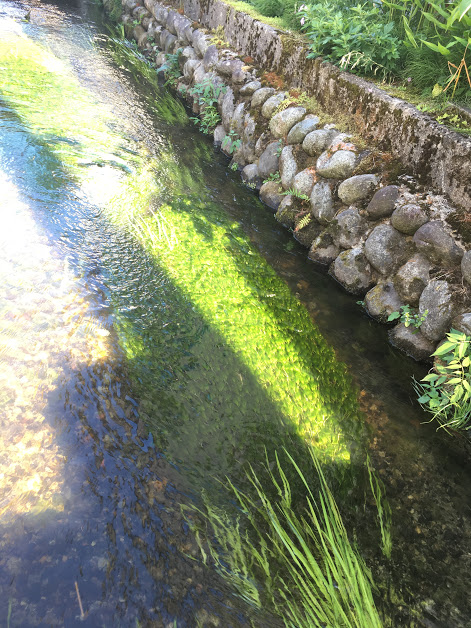(木兰河马大道)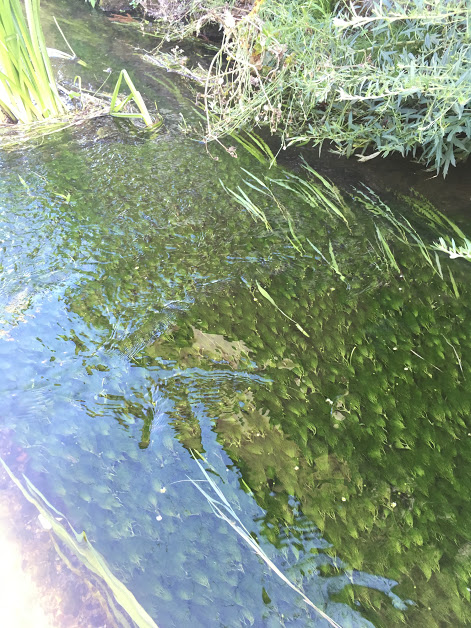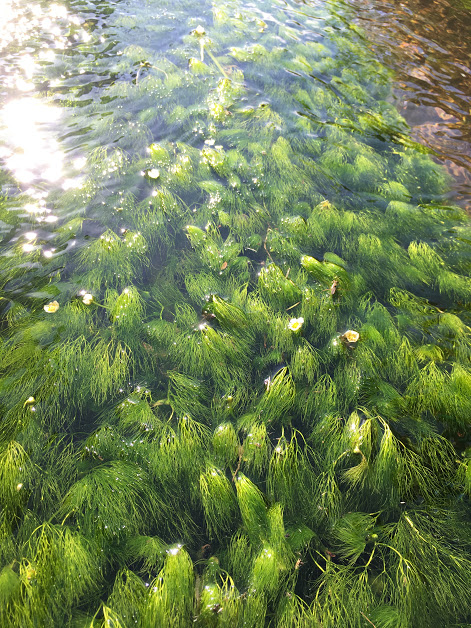(花收成河铃木酒造店长井仓库旁处)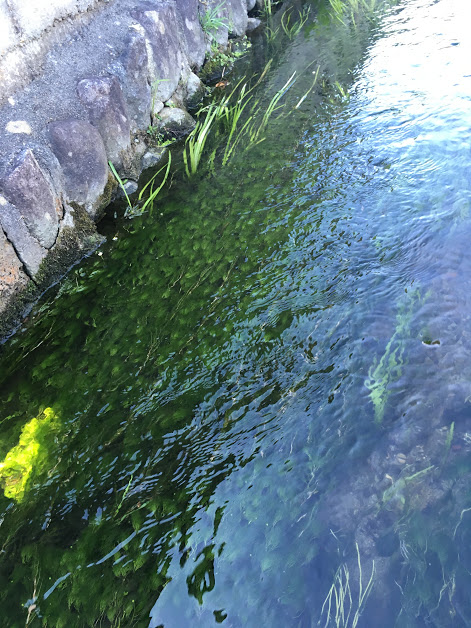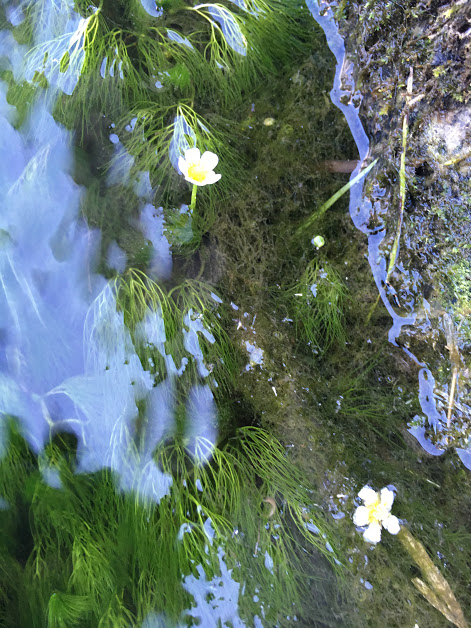(药师寺附近)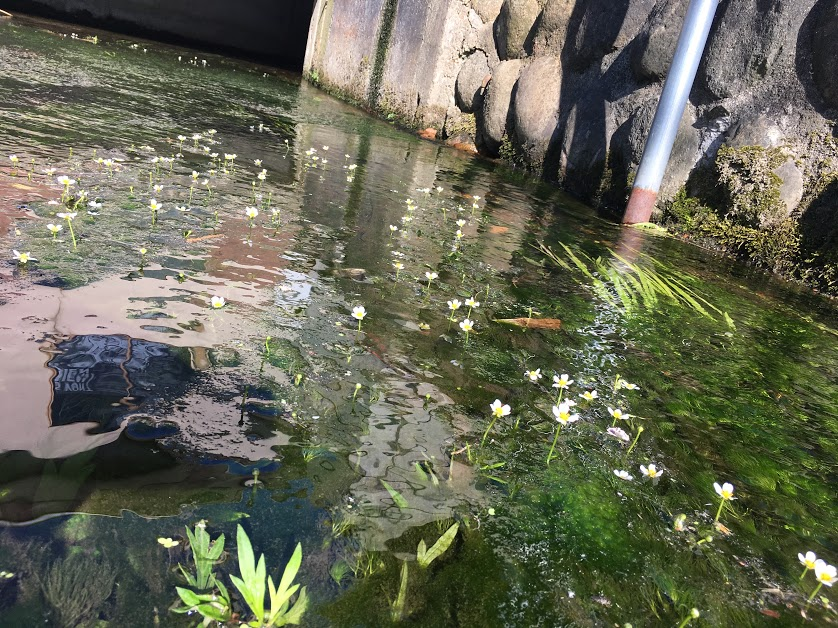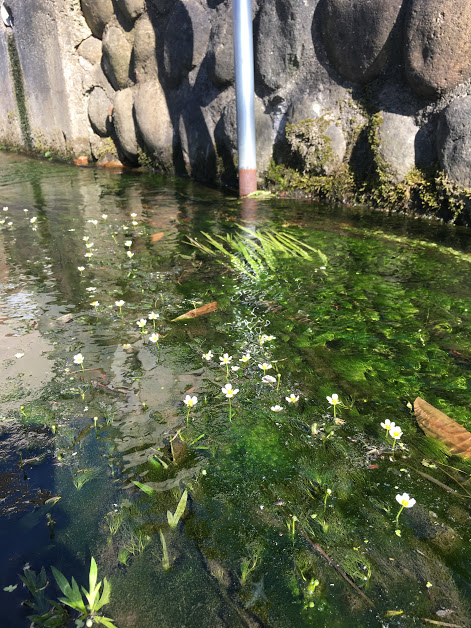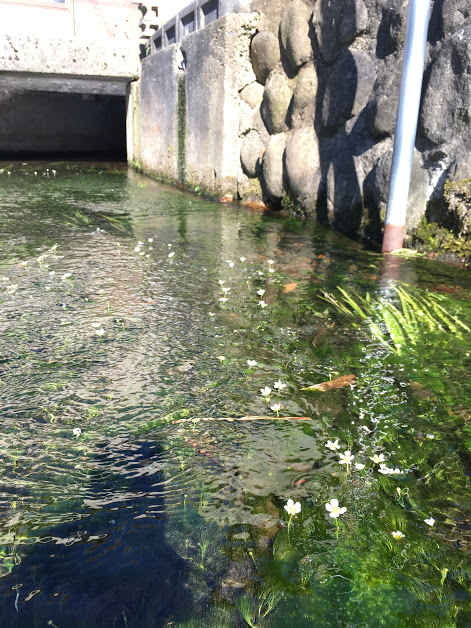(单一田稻荷神社附近)

(2018/7/30拍摄)

=+=+=+=+=+=+=+=+=+=+=+=+=+=+=+=+=+=+=+=+=+=+=+=+=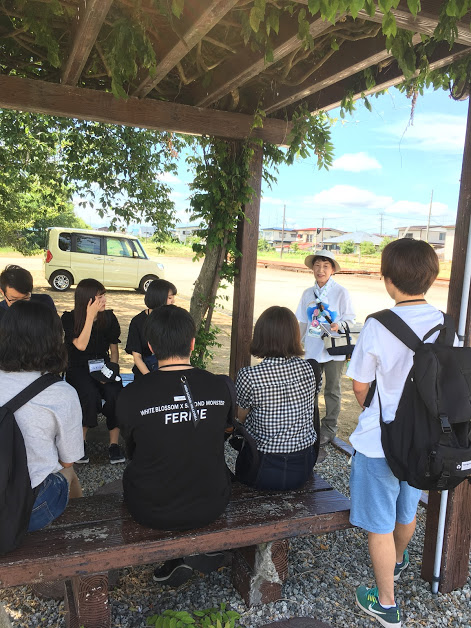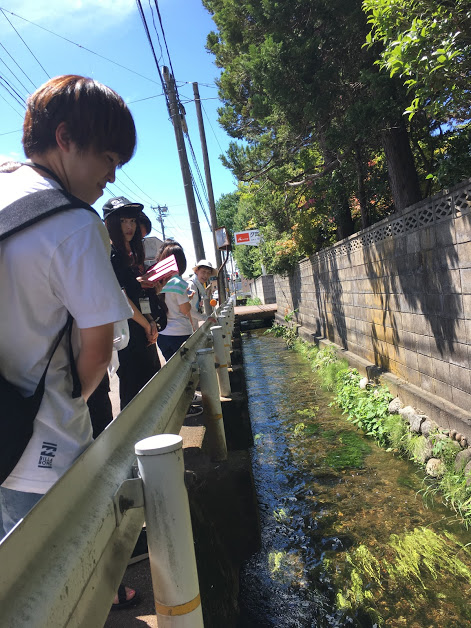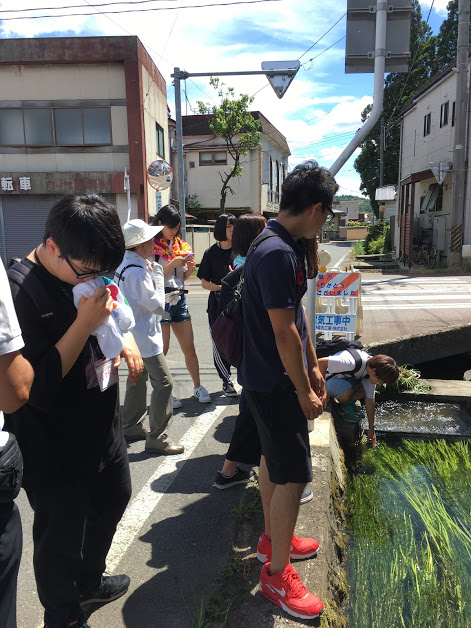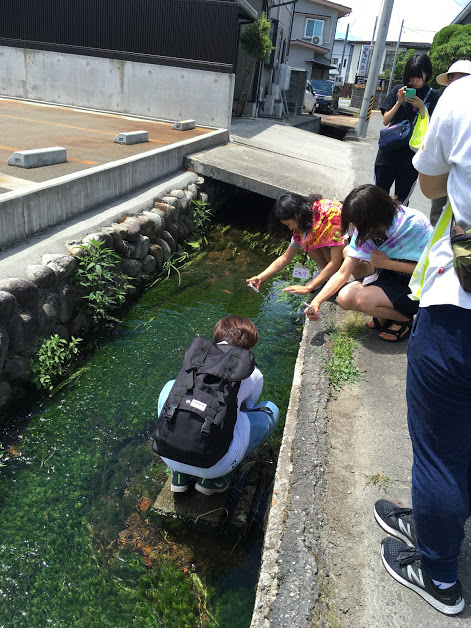2018.07.30

## 对这篇文章的评语是这里

• 名字
• 题名
• 正文
• URL
• ▼供编辑/删掉使用的密码(请在半角英文和数字4个字自由地输入)
• ▼手动输入确认(请用半角英国文字/小写字母输入图片的文字)• 　※任何人投稿可的/立刻，并且反映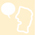### 山形长井观光局|事务局

4,136件合计13,647,127件(2012.01.17-)今天168件昨天